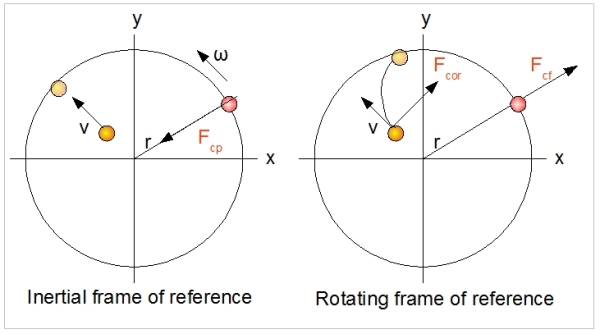# Rotating Reference Frame: Centrifugal and Coriolis Forces

An observer positioned in a rotating reference frame, rotating at a constant angular speed of ω, will observe:

F = m.a + 2mv x ω - m.ω x (ω x r)

where:
F : Force (N)
m : Mass (kg)
v : Speed (m / s)
ω : Angular velocity (rad / s)
r : position vector (m)
x : vector cross product

The component m.a indicates that the Second law of Newton still holds in an rotating reference frame. In fact, a reference frame rotating at a constant, angular speed is also an inertia frame, in which the Newton laws apply.

The component (2m.v x ω) is called the Coriolis force (after the name of the scientist who discovered it).
The component -m.ω x (ω x r) is called the centrifugal force.

Both forces are inertia forces, as their magnitudes depend on the mass, m. The centrifugal force has a direction that is orthogonal to the axis of rotation, and is always directed away from this axis. This Coriolis force is orthogonal to both v and ω. When v=0, the Coriolis force is zero.

## Explanation

In a rotating reference frame, an observer experiences a centrifugal force. One can imagine the existence of this force by realizing that in order for a mass to make a circular motion, a centripedal force of mω2r is needed. When the observer in the rotating reference frame holds a mass m in place, it must, therefore, exercise a force of the same magnitude and in the same direction in order to hold the object in place and at rest (in the moving reference frame). The observer experiences this as a force directed away from the axis of rotation. This force is called the centrifugal force.

Note that we can only speak of a centrifugal force in a rotating reference frame. The centripedal and centrifugal forces are not reaction forces in the sense of the Third Law of Newton, although they have the same magnitude and are opposite in direction. They are forces that exist in different reference frames.

The Coriolis force can be explained as follows. The observer throws a stone away at speed v in the direction of the axis of rotation (see figure x). Because the observer is rotating, and the rock has no real forces working on it, it will not reach point A but point B, because the systems rotate at angular speed ω. So, the observer sees the rock bending away (in the opposite direction of rotation). Since the motion of the stone is curved and hence accelerated, the observer experiences a force which is orthogonal to the speed v of the object. This is called Coriolis force, and will only exist if v>0. The Coriolis force also only exists in a rotating reference frame.

Rotating reference frames play an important role because they simplify reasoning and calculations when looking at rotating systems.## Example

We look again at the example of the rotor that is turning at 500 rpm. We make the assumption that the blades do not have any mass. Instead, all the mass is concentrated at the end of the blades, at one point. This point of mass equals 5 kg. How great is the centrifugal force working on the mass?

Fcf = - m. ω x (ω x r) = -5 . (500 2π / 60)2 . 5 = -68,469 N

Note that the centrifugal forces in a rotor system are highly significant!

# Do you want to comment this topic?

1: (Book) Cyclic and Collective2: (Book) Principles of Helicopter Flight4: Logitech Extreme 3D Pro Joystick5: Saitek Pro Flight Rudder Pedals